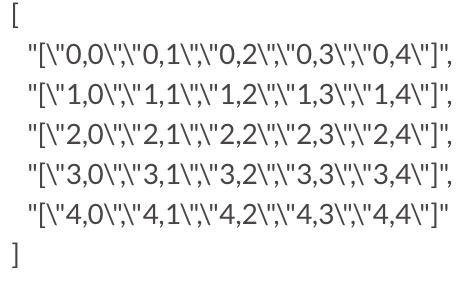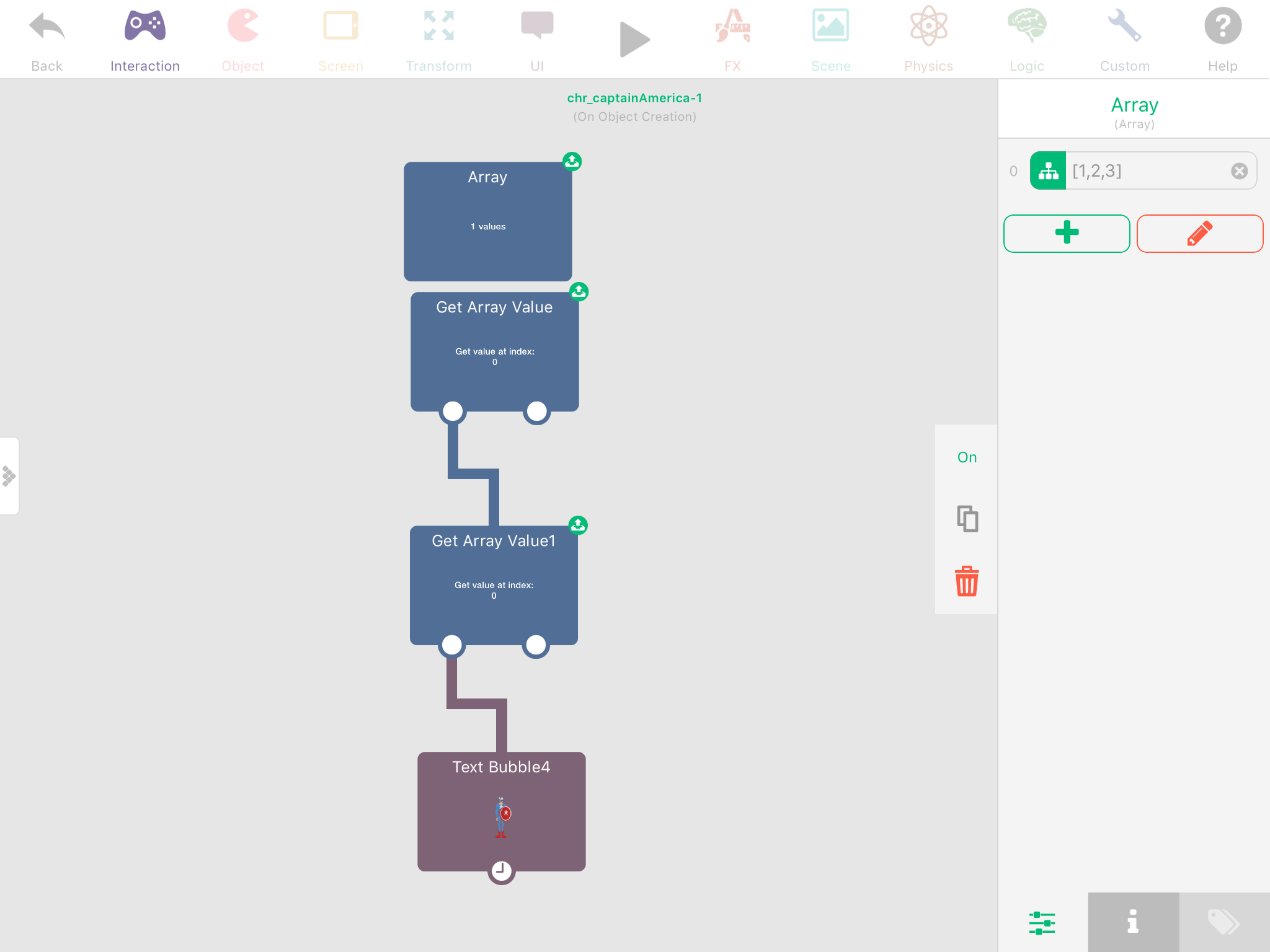Tutorial: how to map a 2-dimensional grid structure/matrix to a 1-dimensional array and back again

• Not sure if this is the best place to post this. Maybe we need a Tutorial section on the forum.

Hyperpad now has array behaviors (yay!), but we’re limited to 1-dimensional arrays. One can do quite a bit with 1-dimensional arrays, but for some things, like grid-based games, 2-dimensional arrays would be ideal. For example, in a game like Battleship, 2-dimensional arrays would be ideal for keeping track of ship locations and the coordinates of hits and misses and then mapping the array information onto a graphic display.

The good news is, we can do the same thing with 1-dimensional arrays, it just takes a few extra steps. So as an example, let’s take a 5x5 grid layout with a ship at coordinates (1,2)(2,2)(3,2) as shown in the left figure.We can assign each square in the grid to an index in a 1-dimensional array with 25 elements as shown in the right figure. Remember, arrays are indexed beginning at 0. So our ship is sitting at array indices 11, 12, & 13.

A 1-dimensional array representation of our ship, where we assign cells with a ship on them a “1” and other cells a “0”, would look like this:

Array = (0,0,0,0,0,0,0,0,0,0,0,1,1,1,0,0,0,0,0,0,0,0,0,0,0)

That is a visual representation of the problem, now how do we actually calculate the array index number for a given grid coordinate? We need one key number – the column dimension or grid width. In this case, we have 5 columns, so the column dimension is 5.

To determine the array index for a given (x,y) coordinate we use the formula:

Array index = (y*column dimension) + x

For the ship at (1,2)(2,2)(3,2), and a column dimension of 5, we calculate:

(1,2) --> (2*5)+1 = 11

(2,2) --> (2*5)+2 = 12

(3,2) --> (2*5)+3 = 13

Which gives us our array indices of 11, 12, and 13.
Pretty cool, huh?

Now how about going the other way. We have our 1-dimensional array representation of the ship, how do we translate that to coordinates so we can then display a graphic representation of the ship in the grid?

This is a little more involved, but not too bad. If we divide an array index by the column dimension, the remainder is our x coordinate and the whole number is our y coordinate.

Let’s take the array index 13 from our previous example. The column dimension is 5. So divide 13 by 5 and get 2 with a remainder of 3, which maps to x=3, y=2.

In Hyperpad we’ll need three behaviors to do this:

Divide Values
Round Number
Modulus

Given an array index “N” and a column dimension “D”

To get x, we use the Modulus behavior which divides two numbers and returns the remainder

x = N mod D

To get y, first divide N by D with a Divide Values behavior,
then input this result into a Round Number behavior, and set the Rounding Type to “Truncate”

y=truncate[N/D]

Hope this makes sense and is helpful.

Note: this could possibly be done with multiple arrays,
but where’s the fun in that! :smiley:

• @mc-games (pretty clever, but multidimensional arrays work)

• @Jack8680 I must be missing something. As far as I understand, the Array behavior in Hyperpad generates a collection of ordered values, hence a 1-dimensional array: Array(i).
How does one create a 2-dimensional array: Array(i,j)?

• @mc-games you can put arrays in arrays. For example in your 5*5 grid, you can have 5 arrays inside an array. It's a bit hard to explain, but here is a 2d array of your grid:
[
"["0,0","0,1","0,2","0,3","0,4"]",
"["1,0","1,1","1,2","1,3","1,4"]",
"["2,0","2,1","2,2","2,3","2,4"]",
"["3,0","3,1","3,2","3,3","3,4"]",
"["4,0","4,1","4,2","4,3","4,4"]"
]

The index of the main array is the X value, then the index of the inside arrays are the y value. For example, if you want the object at 2,4, you would get the array at index 2, which will output the array ["2,0","2,1","2,2","2,3","2,4"]. Then you can get index 4 of that output, which will give the value 2,4. Note that the hyperPad forums are hiding the backslashes in the array, they are automatically created when you use modify array with characters that it might interpret as closing the main array/values:I've also formatted the array, it would actually like like this:
["["0,0","0,1","0,2","0,3","0,4"]","["1,0","1,1","1,2","1,3","1,4"]","["2,0","2,1","2,2","2,3","2,4"]","["3,0","3,1","3,2","3,3","3,4"]","["4,0","4,1","4,2","4,3","4,4"]"]

• @Jack8680 Ahh, arrays within arrays, thanks. This opens up all kinds of possibilities.

• Indeed. I created a project on game press for a chess game. This would be much more realistic now. Thought that project was lost forever, but just found it in my email!• @TutorialDoctor speaking of chess games, I have one I should really finish 😛
http://bit.ly/2oIDvna

• Thanks! for the tutorial and sharing. Right on time for my project.

• @Jack8680 much better than what I had. Please do finish!

• @TutorialDoctor I might eventually, but detecting check/mate would be a bit of effort

• @Jack8680 I guess the theory is to check if all squares around the king are attacked and also to check if any of the pieces are attacking the square the king is on.

• @TutorialDoctor But you would also have to make sure no pieces can defend and no pieces can take the attacking piece for checkmates...